# Influence of a Laser Irradiation and Laser Scribing on Magnetic Properties of GO Silicon Steels Sheets Using a Nanosecond Fiber Laser

Influence of a Laser Irradiation and Laser Scribing on Magnetic Properties of GO Silicon Steels Sheets Using a Nanosecond Fiber Laser

Manar Nesser* Olivier Maloberti Elias Salloum Julien Dupuy Jérôme Fortin

Université de Picardie Jules Verne, Chemin du Thil, Amiens 80025, France

ESIEE-Amiens, 14 quai de la Somme, Amiens 80082, France

Multitel a.s.b.l, Parc Initialis, Mons, BE 7000, France

Corresponding Author Email:
nesser.manar@u-picardie.fr
Page:
439-444
|
DOI:
https://doi.org/10.18280/ejee.230603
30 January 2020
|
Accepted:
10 December 2021
|
Published:
31 December 2021
| Citation

OPEN ACCESS

Abstract:

Improving the performance of electrical steels within the magnetic circuits is essential to save energy. The domain refinement through local surface treatment by laser is an effective technique to reduce the iron losses in grain-oriented iron silicon steels. To interpret the mechanism of this technique, we have quantitatively studied the impact of nanosecond pulse laser treatment on the magnetic properties of grain-oriented Fe(3%wt)Si sheets. We measured the total power loss and apparent permeability of the samples using a Single-Sheet Tester (SST). The laser treatment resulted in a loss reduction of up to 24% compared to the average power loss of standard samples at 50 Hz. At mid-induction levels, the reduction was also accompanied by an improvement in apparent permeability. A dynamic magnetic behavior law was used to identify a dynamic property Λ including information on density, surface area and wall mobility and another internal permeability property µ representative of static field and magnetization characteristics. Lastly, we presented the behavior of these properties under different laser treatment.

Keywords:

coercive field, grain-oriented, irradiation, laser scribing, magnetization properties, silicon steels

1. Introduction

Electrical steels form the magnetic cores of electromagnetic components. Thus, many studies were heading to upgrade these magnetic materials in order to improve their performance in magnetic circuits. The specific magnetic properties required in electrical steel sheets are: a high permeability, a high magnetic saturation and less core loss per cycle. In the present paper we focused on the performance of grain-oriented electrical steels in the rolling direction for power transformers and inductors. These steels have got giant grains that give rise to large magnetic domains. These domains produce losses resulting from the motion of domain walls under the action of an alternative applied magnetic field. These sheets are composed of giant grains that give rise to large magnetic domains. The large domains are responsible for the high dynamic losses resulting from the movement of the domain walls [1, 2]. Thus, a magnetic domain refinement by laser treatment minimizes the speed of wall’s motion and reduces the losses.

Various laser processes have been investigated by researchers: Rauscher et al.  deduced that the use of a pulsed fiber laser on iron silicon plates reduced the loss (average reduction 14.5%) further compared to the use of a continuous CO2 laser (average reduction 12.5%) for the same laser pattern. Similarly, Petryshynets et al.  compared between the impact of a surface treatment with a pulsed laser regime and a continuous laser regime on grains-oriented Fe(3%wt)Si. They achieved higher loss reduction (up to 38%) using the continuous laser. Although, it should be noted that the continuous laser used was of a high power between 12 watts and 30 watts. Moreover, Ponnaluri et al.  performed a surface treatment using an excimer laser on grain oriented silicon steel (M-4 with a thickness of 0.3 mm). They achieved a loss reduction of up to 26%. However, this type of laser generally has a non-homogeneous beam profile and energy, which affects the focus and the reproducibility of the laser tracing.

In this paper, we studied the impact of a local surface laser treatment using a nanosecond fiber laser on the power losses of GO Fe(3%wt)Si. Further, the impact of laser treatment on the apparent permeability of the sheet is important to maintain its performance in magnetic circuits. This criterion has been the subject of additional study. In fact, a laser treatment resulting in loss reduction and a permeability decrease may not be useful because of the additional copper losses required to achieve the same polarization. An optimal laser treatment should be able to reduce power losses without damaging the magnetic permeability. A Single-Sheet-Tester (SST) was used to measure the hysteresis loop of the samples. Then, the variation in loss and apparent permeability of each sample submitted to laser treatment was compared to an average value of 12 standard samples. On the other hand, we used the measured data to identify the internal properties of the dynamic hysteresis model (lambda model):

• $\mu$: representative of the static magnetization;
• $\Lambda$: representative of the domain walls motion.

Finally, the behavior of these internal properties under laser treatment has been studied, analyzed and explained.

2. Methodology

2.1 Model

The magnetic behavior law adopted by Maloberti  presents the magnetic field as a sum of a dynamic field component $\mathrm{H}_{\text {dyn }}$ dependent of a parameter Λ, which is inversely proportional to walls structure, and a static field component $\mathrm{H}_{\mathrm{s}}$ (independent of the electrical conductivity σ and Λ):

$\mathrm{H}=\mathrm{H}_{\mathrm{s}}+\mathrm{H}_{\mathrm{dyn}}$

$\mathrm{H}=\mathrm{H}_{\mathrm{s}}+\sigma \Lambda^{2} \partial_{\mathrm{t}} \mathrm{B}$                   (1)

$\Lambda=\sqrt{\frac{1}{2 \sigma \vartheta \mathrm{J}_{\mathrm{s}} \mathrm{n}_{\omega} \mathrm{m}_{\omega} \mathrm{S}_{\omega}}}$                 (2)

$\Lambda$ (in $\mathrm{m}$ ) is a structural dynamic property summarizing the walls information $\left(s_{\omega}, m_{\omega}, n_{\omega}\right): s_{\omega}$ is the walls surface, $m_{\omega}$ is the walls mobility, and $\mathrm{n}_{\omega}$ is the volume density of walls. Js is the saturation magnetic polarization, $\vartheta$ is a number between 0 and 1 (depending on the polarization direction inside domains).

Then, a calculation sequence starting by the magnetic field diffusion equation led to express the dynamic power loss per unit mass ($\mathrm{P}_{\mathrm{mf}}$) as a function of L with the following equation :

$\mathrm{P}_{\mathrm{mf}}(\Lambda, \omega)=\frac{\omega \xi \mathrm{B}_{\max }^{2}}{4 \mu \mathrm{d}}\left(\frac{\cosh \left(\xi \mathrm{k}_{+}\right)+\cos \left(\xi \mathrm{k}_{-}\right)}{\sinh ^{2}\left(\xi \mathrm{k}_{+}\right)+\sin ^{2}\left(\xi \mathrm{k}_{-}\right)}\right) \times$

$\left(\sigma \Lambda^{2} \mu \omega \mathrm{k}_{+}+\mathrm{k}_{-}\right) \sinh \left(\xi \mathrm{k}_{+}\right)+$

$\left(\sigma \Lambda^{2} \mu \omega \mathrm{k}_{-}-\mathrm{k}_{+}\right) \sin \left(\xi \mathrm{k}_{-}\right)$             (3)

$\mathrm{k}_{\pm}=\sqrt{\frac{1}{2}\left(\frac{\sigma \mu \omega}{1+\left(\sigma \Lambda^{2} \mu \omega\right)^{2}}\right)} \sqrt{\pm \sigma \Lambda^{2} \mu \omega+\sqrt{1+\left(\sigma \Lambda^{2} \mu \omega\right)^{2}}}$

where, $\xi$ is the sheet thickness (sample thickness $\xi=0.23 \mathrm{~mm}$ ), $\mu$ is the internal static permeability characteristic of static magnetic field $\left(\mathrm{H}_{\mathrm{s}}=\mu^{-1} \mathrm{~B}\right)$, a priori independent on the frequency $\mathrm{f}=\omega / 2 \pi$. $\mathrm{d}$ is the mass density of steel. $\mathrm{B}_{\max }$ is the peak induction of each hysteresis cycle. The average magnetic induction within the sample cross section is given by the equation:

$\langle B\rangle=\frac{2 \mu \mathrm{H}_{\mathrm{a}}}{\xi} \frac{\tanh \left(\left(\mathrm{k}_{+}+\mathrm{jk}_{-}\right) \xi / 2\right)}{\left(1+\mathrm{j} \sigma \Lambda^{2} \mu \omega\right)\left(\mathrm{k}_{+}+\mathrm{jk}_{-}\right)}$             (4)

$\langle B\rangle=B_{\max } \cos (\omega t+\varphi)$                  (5)

Ha is the magnetic field applied onto the sheet surface.

At time $t=\frac{-\varphi}{\omega}:\langle B\rangle=B_{\max }$ and $H_{\max }=H_{a} \cos \varphi$.

The apparent permeability is then expressed as:

$\mu_{\mathrm{app}}=\frac{\mathrm{B}_{\max }}{\mathrm{H}_{\max }}=\frac{\mathrm{B}_{\max }}{\mathrm{H}_{\mathrm{a}} \cos \varphi}$              (6)

$\Lambda$ is defined with Eq. (2) and $\mu$ is defined by the local law $\mathrm{H}_{\mathrm{s}}=\mu^{-1} \mathrm{~B}$. To determine the values of $\Lambda$ and $\mu$, we used the data measured with SST to deduce the dynamic component of the total power loss and the apparent permeability. Knowing the value of $P_{\mathrm{mf}}$ and $\mu_{\mathrm{app}}$ at specified frequency $f$ and peak induction $B_{\max }$, we finally solved the system of two equations (3) and (6) with two unknowns to find the values of $\Lambda$ and $\mu$.

2.2 Sample material and laser process

The material tested is a grain-oriented silicon iron SiFe sheet (dimensions: 150×150×0.23 mm3) insulated with a coating of thickness equal to 0.002 mm. The density of the sheet is equal to 7380 kg.m-3.

The samples were treated with an IPG pulsed Ytterbium fiber nanosecond laser that delivers a high power 1.064 µm radiation. The average power used during treatment is between 0.5 W and 10 W for different laser pulse width between 4 ns and 200 ns. Laser lines are scanned on both sides of the sheet with patterns of parallel lines spaced by 3 mm in a direction perpendicular to the rolling direction with a constant spot size of 50 µm. To present the results, we have classified the laser configurations into two categories according to their effect on the surface of the sample:

- Laser irradiation: the effect of the laser is mainly a thermal heating of the surface without any significant relief formation or deformation.

- Laser scribing: the tracing of the laser line sinks a few micrometers into the surface of the sample with a formation of a small relief around the groove.

It should be mentioned that laser irradiation can cause a change in the internal stress induced by thermal heating , and that a simultaneous thermal effect can also occur for laser scribing, especially with longer pulses . The thermal induced stress study is under investigation and will be developed elsewhere.

3. Results

The hysteresis loop of each sheet is measured with a Single Sheet Test System (SST). It adjusts the suitable value of the applied magnetic field Ha to reach the requested induced magnetic flux for specified frequency. The measurements were performed with a sinusoidal waveform. The external magnetic field is applied on the sheet surface along its rolling direction. We presented the power loss, apparent permeability variations, besides the two parameters (internal parameters Λ and µ) at low (0.1T) and high induction (1.5T) for different frequencies and under different laser treatments.

The initial value at each frequency and induction level is the average value of 12 standards samples.

The confidence interval is presented on the graphs below; it represents the 95% confidence interval (using a normal distribution law) calculated with the following expression:

$\mathrm{I}_{\mathrm{c}}=\left[\overline{\mathrm{x}}-(1.645) * \frac{\mathrm{s}}{\sqrt{\mathrm{n}}} ; \overline{\mathrm{x}}+(1.645) * \frac{\mathrm{s}}{\sqrt{\mathrm{n}}}\right]$             (7)

where, $\bar{X}$ is the mean value, s is the standard deviation and n the number of samples. This confidence bar includes the dispersion between standard samples, the accuracy of the SST equipment and the accuracy of the sinusoidal measurement.

3.1 Identification of dynamic magnetization property Λ and internal static permeability µ

We studied the behavior of the dynamic magnetization property Λ as a function of the induction level for frequency 50 Hz. The result presented in Figure 1 showed a decrease in the value of Λ with the increase in induction level to achieve a minimum value at mid-induction level (~ 1 T). Then Λ increased again at higher induction level.

## 1.png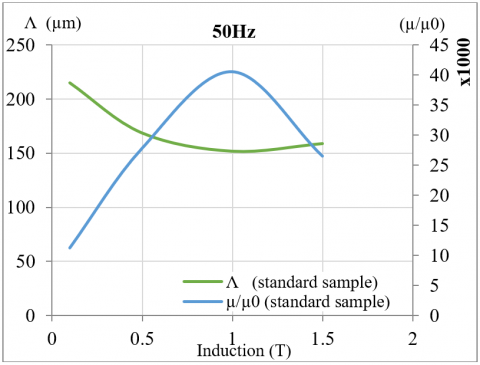Figure 1. The values of Λ and µrel of standard sample as a function of the induction level at 50 Hz

## 2.pngFigure 2. The values of Λ and µrel of standard sample as a function of the frequency at 1.5 T

This refers to the increase in either walls density, surface or mobility at mid-induction then to the decrease of walls density near saturation at high induction. The behavior of the internal permeability m was symmetrical to the behavior of Λ as a function of induction level (Figure 1 @50 Hz). It showed an increase by increasing the induction level to achieve a maximal value at mid-induction level (~ 1 T), then m decreased again at higher induction level. At low induction, i.e. in the Rayleigh zone, the permeability is reduced by the walls pinning effect on the defects at low field. At high induction, the permeability m is reduced by demagnetizing effects due to magnetic poles created within the saturation zone. The permeability is thus optimized at mid-induction between the Rayleigh zone and the saturation.

The value of Λ for a fixed induction (1.5 T), as shown in Figure 2, decreased as a function of the frequency because the walls mobility, surface and density may increase by increasing the frequency of the applied field. This is mainly due to magnetization reversal mechanisms such as the domains’ walls bowing and multiplication. This behavior is similar whatever the induction level.

As expected, the behavior of the internal static permeability m did not change significantly as a function of the frequency at high induction level (Figure 2).

3.2 Relative influence of surface laser treatments on the properties Λ and µ

From Figure 3, we concluded that the optimum value of Λ and the corresponding induction level depended on the laser configuration used during the treatment process (laser scribing configuration with short pulse: optimum induction level 0.5 T with a reduction of 9 % (±2.38%) in Λ @50 Hz).

For the same frequency 50 Hz, the maximum increase in µ was 23 % (±3.36%) at 0.5 T also for the sample scribed with the short pulse laser (Figure 4).

## 3.png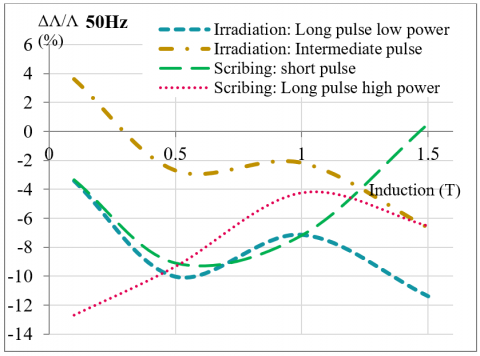Figure 3. Variation of the dynamic magnetization property Λ of treated samples as function of the induction level at 50 Hz (precision on calculation ~ ±2.38%)

## 4.png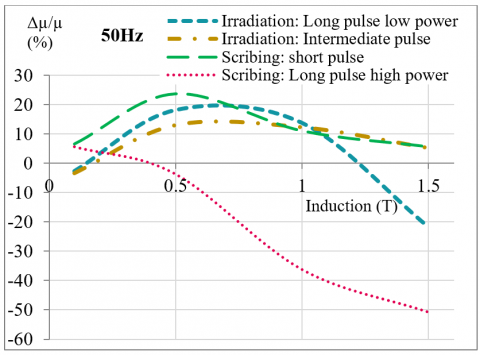Figure 4. Variation of the internal static permeability µ fig of treated samples as function of the induction level at 50 Hz (precision on calculation ~ ±3.36%)

The Figures 5 and 6 presented the variation of the dynamic magnetization property lambda as function of the frequency at high induction (1.5T) and low induction level (0.1T) respectively. The laser scribing configuration with short pulse and the laser irradiation with long pulse and low power resulted both in a maximum decrease in the value of Lambda at high induction while this reduction was accompanied by an increase in the internal static permeability µ only in the case of laser scribing with short pulse width (Figure 7). The maximum impact on Lambda appeared at frequencies between 50 and 100 Hz for high inductions and for higher frequencies at low inductions. The behavior of the internal static permeability was similar to the apparent permeability. At low induction level, a laser scribing with longer pulse decreased Lambda Λ the most with a maximum of 16 % and increased µ up to a maximum of 14 % (Figure 8). The decrease in the values of Λ after laser treatment refers to the domain walls refinement process, which results mainly in an increase of the walls density.

## 5.png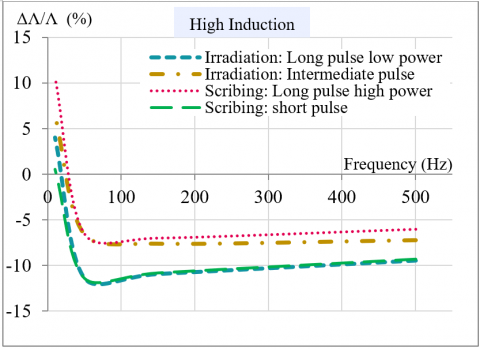Figure 5. Variation of the dynamic magnetization property Λ of treated samples as a function of frequency at high induction level (1.5T) (precision on calculation ~ ±2.38%)

## 6.png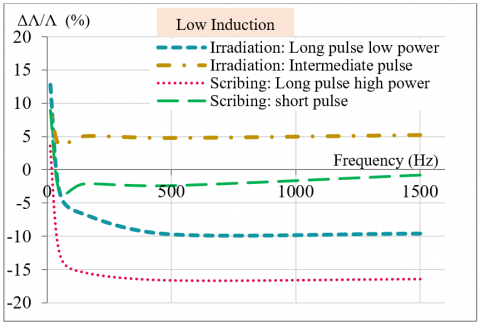Figure 6. Variation of the dynamic magnetization property Λ of treated samples as a function of frequency at low induction level (0.1T) (precision on calculation ~ ±2.38%)

In some cases, the laser mark could produce walls nucleation centers at high frequency that in his turn increase the walls density (walls nucleation and multiplication). Also the value of µ increased after laser treatment for some laser configurations. We expected a decrease in the apparent permeability after laser treatment especially in the case of laser scribing because laser mark forms an artificial edge of grain or some defects that may pin the walls, but we found the opposite. The reason behind this improvement could refer to the pre-stress present in the sample (due to the coating process) that is released by the thermal stress imposed by the laser treatment also by the damaged coating.

## 7.png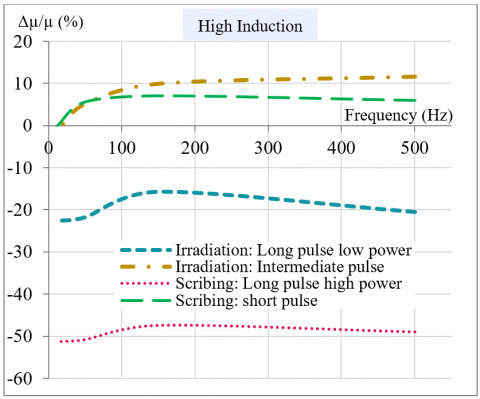Figure 7. Variation of the internal static permeability µ of treated samples as a function of frequency at high induction level (1.5 T) (precision on calculation ~ ±3.36%)

## 8.pngFigure 8. Variation of the internal static permeability µ of treated samples as a function of frequency at low induction level (0.1T) (precision on calculation ~ ±3.36%)

3.3 Results on the total power and apparent permeability improvements

SST measurement results at high induction level, presented in Figure 9, showed that the best reduction of total power loss occurred in the case of sample submitted to laser scribing with short pulse and sample submitted to laser irradiation with long pulse and low power. However, these two laser configurations had opposite impacts on the apparent permeability of the samples; the laser scribing with short pulse resulted in a slight increase of the apparent permeability (maximum value 5%) while the laser irradiation strongly decreased the apparent permeability up to 20%. Thus, the best power loss reduction recorded for the sample treated with a short laser pulse @50 Hz was 20.5% accompanied with slight increase in the apparent permeability as shown in Figure 11. The error bars in the graphs includes the dispersion between standard samples which was quite high (~14%), the accuracy of the SST equipment (between 1% and 2%) and the accuracy of the sinusoidal measurement (high for induction level > 1.2 T).

## 9.png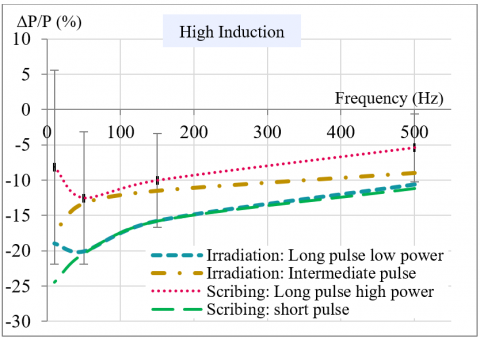Figure 9. The variation of the total power losses of treated samples as a function of frequency at high induction level

## 10.png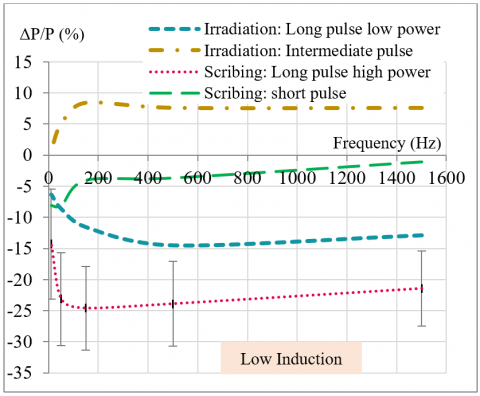Figure 10. Variation of the total power losses of treated samples as a function of frequency at low induction level

## 11.png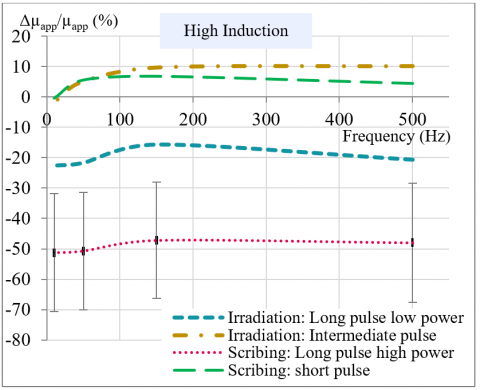Figure 11. Variation of the apparent permeability of treated samples as a function of frequency at low induction level

At low induction level, a power loss reduction between 13% and 23% occurred for sample scribed with long pulse and high power (Figure 10). This configuration showed also an improvement in the apparent permeability up to 13% (Figure 12). Although, the laser irradiation showed a reduction in the total power loss between 6% and 13%, this configuration decreased the apparent permeability with a value up to 8%. Accordingly, Figure 13 shows that short-pulse laser processing can reduce total power losses and improve the apparent permeability of a grain-oriented sheet at high induction for a frequency of 50 Hz. Whereas a long pulse laser treatment is useful to minimize these losses at high frequencies for an induction of 0.1 T (see Figure 14).

## 12.pngFigure 12. Variation of the apparent permeability of treated samples as a function of frequency at low induction level

## 13.png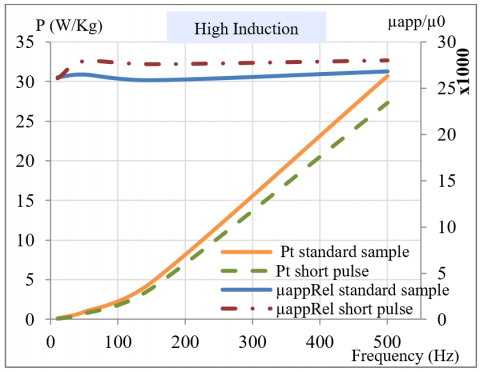Figure 13. The total power losses and the relative permeability as a function of frequency at high induction level (1.5 T)

## 14.png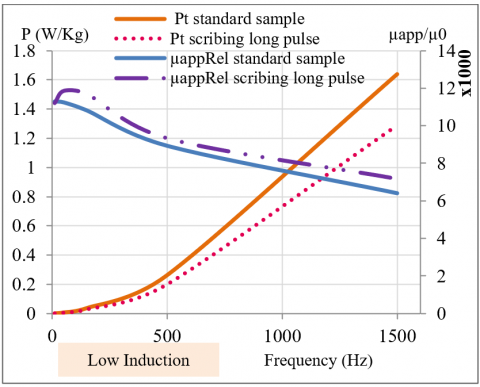Figure 14. The total power losses and the relative permeability as a function of frequency at low induction level (0.1 T)

We deduced that the configuration of laser irradiation which reduced the power loss damaged the apparent permeability while the laser scribing reduced the power loss and improved the apparent permeability. The improvement in the apparent permeability could refer to the local damage of the coating that released locally the residual stress resulting from the coating process.

4. Conclusions

To summarize, we showed that a short pulse laser treatment is recommended to reduce the total power losses of grain-oriented sheet at high induction level especially for frequency 50 Hz (operating frequency of electrical machines) while a laser scribing with long pulse and high power is useful to reduce these losses at low induction level for high frequencies (harmonic frequencies).

Also, we concluded that the laser scribing with short pulse improved the apparent permeability. Noting that in this study we distinguished between the laser scribing and laser irradiation because each one behaves differently on the sample’s coating layer; the irradiation process doesn’t affect significantly the coating layer while the laser scribing damages it partly thus an insulating process is needed after laser scribing maintaining the loss reduction and the in the apparent permeability increase. In the future studies further investigations in the correlation between different laser parameters and these magnetic properties are required to control the laser process.

Acknowledgment

This work was carried out within the frame of the project ESSIAL, funding from the European Union's Horizon 2020 research and innovation program grant agreement No 766437.

Nomenclature
 $\langle B\rangle$ average magnetic induction, T f frequency, Hz $\mathrm{H}_{\mathrm{a}}$ applied magnetic field, A/m $\mathrm{H}_{\mathrm{s}}$ static magnetic field, A/m $I_{c}$ confidence interval $\mathrm{J}_{\mathrm{S}}$ saturation magnetic polarization, T $\mathrm{m}_{\omega}$ walls mobility, µm/s n number of samples $\mathrm{n}_{\omega}$ volume density of walls, µm-2 $\mathrm{P}_{\mathrm{mf}}$ dynamic power loss per unit mass, W/kg s standard deviation $S_{\omega}$ walls surface, µm2 t time, s $\bar{X}$ mean value Greek symbol $\xi$ sheet thickness, m $\mu$ static internal permeability, H/m $\Lambda$ dynamic magnetization property, µm $\sigma$ electrical conductivity, (Ω.m)-1 $\vartheta$ number between 0 and 1 $\varphi$ magnetic flux, Wb $\omega$ angular velocity, rad/s
References

 Overshott, K. (1976). The use of domain observations in understanding and improving the magnetic properties of transformer steels. IEEE Transactions on Magnetics, 12(6): 840-845. https://doi.org/10.1109/TMAG.1976.1059118

 Patri, S., Gurusamy, R., Molian, P.A., Govindaraju, M. (1996). Magnetic domain refinement of silicon-steel laminations by laser scribing. Journal of Materials Science, 31(7): 1693-1702. https://doi.org/10.1007/BF00372180

 Rauscher, P., Hauptmann, J., Beyer, E. (2013). Laser scribing of grain oriented electrical steel under the aspect of industrial utilization using high power laser beam sources. Physics Procedia, 41: 312-318. https://doi.org/10.1016/j.phpro.2013.03.083

 Petryshynets, I., Kováč, F., Puchý, V., Šebek, M., Füzer, J., Kollár, P. (2018). Magnetic losses reduction in grain oriented silicon steel by pulse and continuous fiber laser processing. AIP Advances, 8(4): 047604. https://doi.org/10.1063/1.4994191

 Ponnaluri, S.V., Cherukuri, R., Molian, P.A. (2001). Core loss reduction in grain-oriented silicon steels by excimer laser scribing: Part I: experimental work. Journal of Materials Processing Technology, 112(2-3): 199-204. https://doi.org/10.1016/S0924-0136(01)00573-8

 Maloberti, O. (2006). Contribution à la modélisation de la dynamique d'aimantation dans les matériaux magnétiques doux: caractérisation et simulation (Doctoral dissertation, Institut National Polytechnique de Grenoble-INPG).

 Maloberti, O., Kedous-Lebouc, A., Geoffroy, O., Meunier, G., Mazauric, V. (2006). Field diffusion-like representation and experimental identification of a dynamic magnetization property. Journal of Magnetism and Magnetic Materials, 304(2): e507-e509. https://doi.org/10.1016/j.jmmm.2006.02.260

 Li, J., Gu, Y., Guo, Z. (1997). Decreasing the core loss of grain-oriented silicon steel by laser processing. Journal of Materials Processing Technology, 69(1-3): 180-185. https://doi.org/10.1016/S0924-0136(97)00015-0

 Petryshynets, I., Puchý, V., Kováč, F., Šebek, M. (2017). Effect of laser scribing on soft magnetic properties of conventional grain-oriented silicon steel. Acta Physica Polonica A, 131(4): 777-779. https://doi.org/10.12693/APhysPolA.131.777